Courses

# Sequences And Series Of Real Numbers -1

## 20 Questions MCQ Test Topic-wise Tests & Solved Examples for IIT JAM Mathematics | Sequences And Series Of Real Numbers -1

Description
This mock test of Sequences And Series Of Real Numbers -1 for Mathematics helps you for every Mathematics entrance exam. This contains 20 Multiple Choice Questions for Mathematics Sequences And Series Of Real Numbers -1 (mcq) to study with solutions a complete question bank. The solved questions answers in this Sequences And Series Of Real Numbers -1 quiz give you a good mix of easy questions and tough questions. Mathematics students definitely take this Sequences And Series Of Real Numbers -1 exercise for a better result in the exam. You can find other Sequences And Series Of Real Numbers -1 extra questions, long questions & short questions for Mathematics on EduRev as well by searching above.
QUESTION: 1

### ​The sequence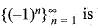Solution: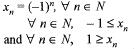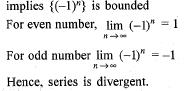QUESTION: 2

### The series 1 · x + 1 · 2x2 + 1 · 2 3x2 + ... + + ... + n! · xn + ... is

Solution:

Explanation : an = n!

then |an+1/an| = (n+1)/n!

= n+1

L = lim(n-->∞) |an+1/an|

= ∞

The radius of convergence R = 0 Hence, the series converges only at x = 0.

Option A) is correct, it is divergent.

QUESTION: 3

### The series 2 + 4 + 6 + 8 + ...is

Solution: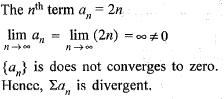QUESTION: 4

The scries x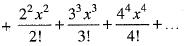is convergent, if

Solution:

If ∑μn be the given series, then we have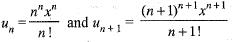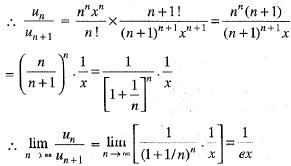∴ From ratio test, the given series ∑μis convergent or divergent according as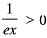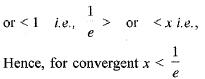QUESTION: 5

If a1, a2,  a3,  ... be a sequence of non-zero integers satisfying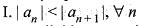II. an divides an + 1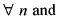III. every integer is a divisor of some an, then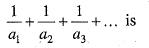Solution: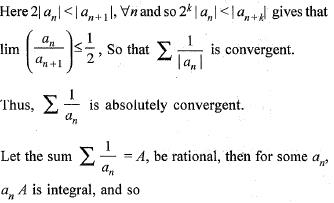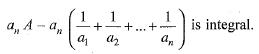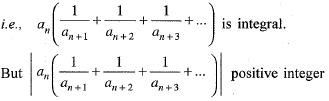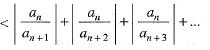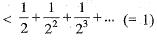is a contradiction chair, and so, A is an irrational number.

QUESTION: 6

Let ∑μn be a series of positive terms. Given that ∑μn is convergent and also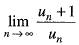exists, then the said limit is

Solution: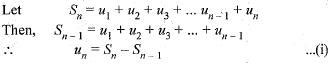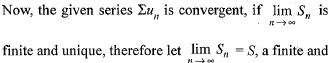unique quantity.
Then, from Eq. (i) we have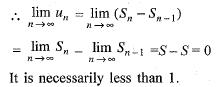QUESTION: 7

The Series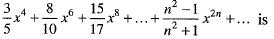Solution:

Here, given series is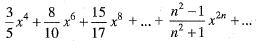∴ If ∑μn be the given series, then we have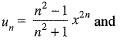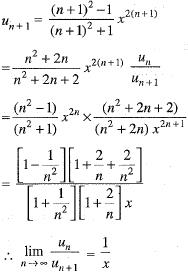Therefore, from ratio test, we conclude that the given series ∑μn is convergent or divergent according as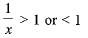i. e., according as x < 1 or > 1 ...(i)
If n = 1, this test fails and the given series reduces to
∑μwhose nth term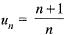and can be proved to be divergent.
Hence, we conclude that the given series is convergent, if x2 ≤ 1 and divergent, if x2 ≥ 1.

QUESTION: 8

The sequence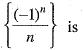Solution:

We have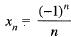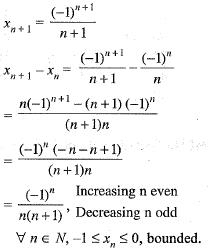QUESTION: 9

The sequence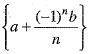Solution: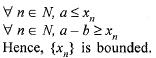QUESTION: 10

Match list I with list II and select the correct answer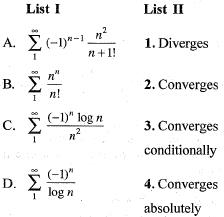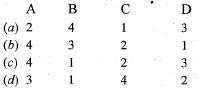Solution: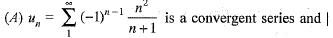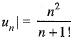is also convergent series.
∴ un is also convergent series.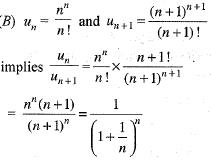Hence, by d’Alembert test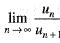implies un is divergent series.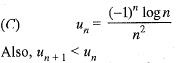By Cauchy condensation test, ∑μn is convergent series.
(D)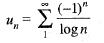By Leibnitz’s test, the series is convergent.
Also,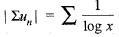is divergent series.
So, the given series is converges conditionally.

QUESTION: 11

The series whose nth term is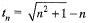Solution:

Here it is given that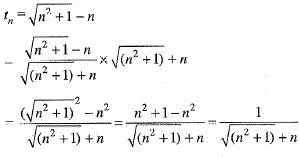Now, taking auxiliary series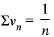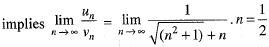Which is finite and non-zero. Since, vn is divergent.
Hence, tn is also divergent.

QUESTION: 12

The series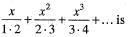convergent, if

Solution:

Here given series is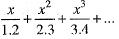is convergent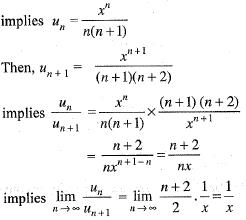∴ The given series will be convergent, if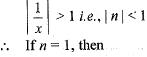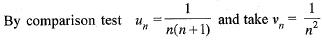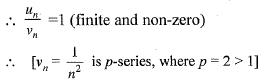Hence, vn is convergent series, so un is also convergent.
∴ The given series is convergent, if | x | ≤ 1.

QUESTION: 13

The series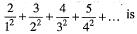Solution:

Let the given series be denoted by ∑μn, then
∑|μn|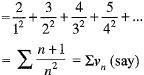Compare this series ∑vn with the auxiliary series,Then,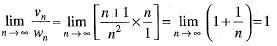which is finite quantity.
Hence, ∑un and ∑wn are either both convergent or both divergent. But ∑wn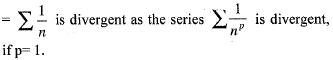Hence, the series ∑vn is divergent.
Also, in the series ∑un, we find that its term are alternately positive and negative, its terms are continuously decreasing and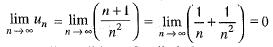Thus, all condition of Leibnitz’s test are satisfied and as such ∑un is convergent. H ence, the given series ∑un is conditionally convergent.

QUESTION: 14

The series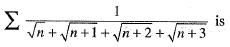Solution: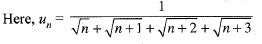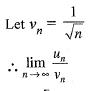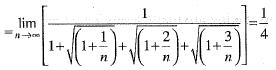which is finite and non-zero.
Since, ∑vn is divergent, therefore, ∑un is also divergent.

QUESTION: 15

The series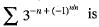Solution: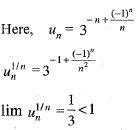Hence, by Cauchy’s root test, the series convergent.

QUESTION: 16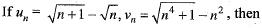Solution:

Here, it is given that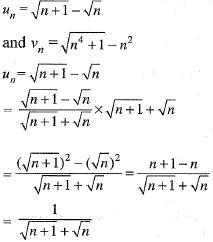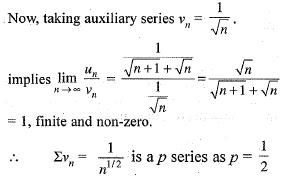Hence, ∑vn is divergent and ∑un is also divergent and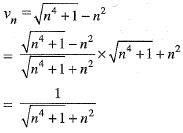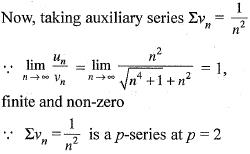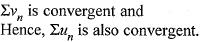QUESTION: 17

The series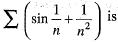Solution:

Here,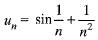Let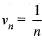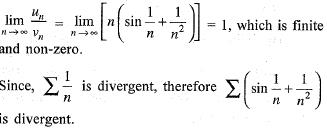QUESTION: 18

The series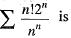Solution: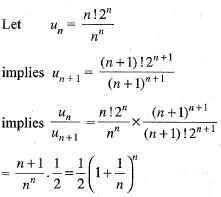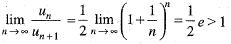Hence, by d’Alembert’s ratio test, it is convergent.

QUESTION: 19

The series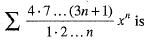convergent, if

Solution: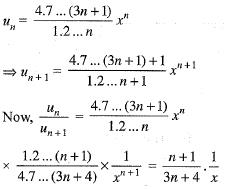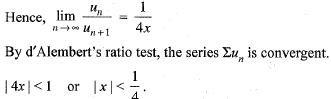QUESTION: 20

The series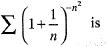Solution:

The nth term of the given series is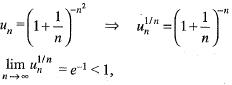Hence, ∑un is convergent.